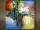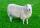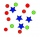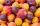# Candies

There are red, blue and green candies in bad. Red to green is in 6:11 ratio and blue to red in a 7: 5 ratio. In what proportion are blue to green candies?

Result

p =  42:55

#### Solution:Leave us a comment of example and its solution (i.e. if it is still somewhat unclear...):

Showing 0 comments:Be the first to comment!#### To solve this example are needed these knowledge from mathematics:

Need help calculate sum, simplify or multiply fractions? Try our fraction calculator. Most natural application of trigonometry and trigonometric functions is a calculation of the triangles. Common and less common calculations of different types of triangles offers our triangle calculator. Word trigonometry comes from Greek and literally means triangle calculation.

## Next similar examples:

1. PeppersIn the box are yellow (a), green (b) and red (c) peppers. Their amount is in a ratio 2:4:1 . Most are yellow peppers and green the least. Calculate the number of peppers each type if the total number of peppers is 70.
2. Land areaA land area of Asia and Africa are in a 3: 2 ratio, the European and African are is 1:3. What are the proportions of Asia, Africa, and Europe?
3. Image scaleThe actual image dimensions are 60 cm x 80cm and has a reduced size 3 cm x 4 cm. At what scale the image was reduced?
4. RatiosReduce the numbers: 50 in a 1:2 ratio 111 at a ratio of 2:3 70 at 10:50 560 at a ratio of 3:8
5. DonutsFind how many donuts each student will receive if you share 126 donuts in a ratio of 1:5:8
6. Three 43Three brothers inherited a cash amount of 62,000 and they divided it among themselves in the ratio of 5:4:1. How much more is the largest share than the smallest share?
7. Line segmentThe 4 cm long line segment is enlarged in the ratio of 5/2. How many centimeters will measure the new line segment?
8. AgesJohn, Teresa, Daniel and Paul have summary 56 years. Their ages are in a ratio of 1:2:5:6. Determine how many years have each of them.
9. The farmerThe farmer had 140 sheep. For the next year, she decided to change the number of sheep in ratio 10: 7. How many sheep will he have then?
10. Divide 5Divide 288 in the following ratio 3 : 4 : 5
11. BuildingAt the building, we divided 240 boards into two piles in a 5: 3 ratio. How many were fewer boards in the lower pile?
12. Divide moneyDivide 1200 USD at a ratio of 1:2:3:4:5:6:9:10
13. RunnersFor three runners (on the first to third place) is prepared 30 chocolate that they be distributed in the ratio of 3 : 2 : 1 How much chocolate will get everyone?
14. AndreAndre, Thomas, and Ivan split 88 postage stamps in a 2:5:4 ratio. How much did Thomas get?
15. Proportion 2A car is able to travel 210 km in 3 hours. How far can it travel in 5 hours? Put what kind of proportion is this and show your solution.
16. HomeworkIn the crate are 18 plums, 27 apricot and 36 nuts. How many pieces of fruit left in the crate when Peter took 8 ninth: 1. nuts 2. apricots 3. fruit 4. drupe
17. Equation with xSolve the following equation: 2x- (8x + 1) - (x + 2) / 5 = 9